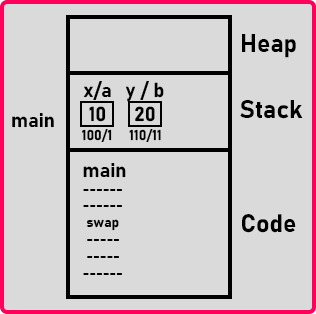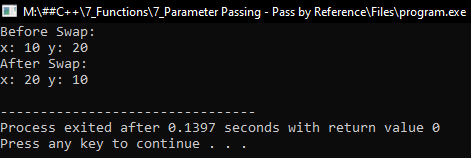# Parameter Pass by References in C++

## Parameter Pass by References in C++ with Examples:

In this article, I am going to discuss Parameter Pass by References in C++ Language with examples. Please read our previous article, where we discussed Parameter Pass by Address in C++ with examples.

##### Parameter Pass by References in C++:

References are the nickname to an existing variable. So let us look at called by reference mechanism in C++ with an example.

void Swap(int &a, int &b){
int temp;
temp = a;
a = b;
b = temp;
}
int main(){
int x = 10, y = 20;
Swap(x, y);
cout << x << ” ” << y;
}

Here we have written ‘&’ before variables names “void Swap(int &a, int &b)”. So, the syntax of writing is the same as call by value but only the change is, we have written ‘&’ at the parameters and they become references.

But now this mechanism can modify the actual parameters. It works similarly to the call-by address. So unlike call by value where the changes cannot be done in actual parameters but in the call by reference changes can be done in the actual parameters.

##### How does Pass by References works in C++?

Let’s see how it works. First the main function start, the moment the main function start, the variables are created that is ‘x’ is having ‘10’ and ‘y’ is having ‘20’. Then ‘swap’ function is called. This function is having three variables that are a, b and temp. But what are ‘a’ and ‘b’? They are not separate variables; they are references to ‘x’ and ‘y’.

So how it is possible that the ‘swap’ function can directly access the variables of the ‘main’ function? It’s not possible. Then how does it happens? When we use a call by reference mechanism, the main function is having its code and in this place that is where the swap function is called the machine code of the swap function will be copied.So, this means that whenever you use call by reference mechanism, it will not generate a separate piece of machine code. It will copy the machine code at the place of the function call. So, this swap is not a separate function. It’s a part of the main function only.

So, there is no activation record created for the swap function and actually, the swap function is not called. There is no function at all in machine code. In source code, we wrote function but in machine code, there is no function. Then what about this temp.

##### What about the temp variable?

The temp will also be created for time being inside the activation record of the main function only. And this will be there as long as this piece of swap code is executing.

This is the greatness of C++ that is no other language having this type of feature that basically whenever you write a separate function a separate piece of machine code will be generated. But in C++ that piece of machine code can be replaced at the place of the function call.

##### When to use call by reference?

Whenever we want the actual parameters should be modified. The second point you should not write any complex logic inside the function if you are using call by reference.

The reason is, the function code has to be copied inside the main’s machine code whatever the function is called if you call multiple times then multiple times copying will be done. So, when the code is being copied, this is the job of a compiler to copy the code there. If the code is complex, it may not be able to do it perfectly.

Usually, you find warnings if you’re using loops inside this type of function. When you use call by reference avoid using the loop. One or two lines of the statement are sufficient.

Now one more point here is that if the piece of machine code of a function is copied at the place of a function call, then such functions are called inline functions in C++. When you use a call by reference function automatically it becomes an inline function. We will discuss the inline function in upcoming articles in more detail. Now let us write a complete program for this.

##### Parameter Pass by References Program using C++:
```#include <iostream>
using namespace std;

void Swap(int &a, int &b)
{
int temp;
temp = a;
a = b;
b = temp;
}

int main()
{
int x = 10, y = 20;
cout << "Before Swap:\n";
cout << "x: " << x << " y: " << y << endl;
swap (x, y);
cout << "After Swap:\n";
cout << "x: " << x << " y: " << y << endl;
}
```
###### Output:#### Parameter Passing Frequently Asked Questions

##### How does Call by Reference work?

In call by reference, the compiler may make a function as inline. The machine code of the function may be copied at the place of the function call.
Or
The compiler may convert the reference into a constant pointer. (constant pointer: a pointer is initialized once and cannot be changed)

##### What happens, If one parameter is a reference and another pointer?

Obviously, the function will not become an inline function. The compiler will convert a reference into a constant pointer.